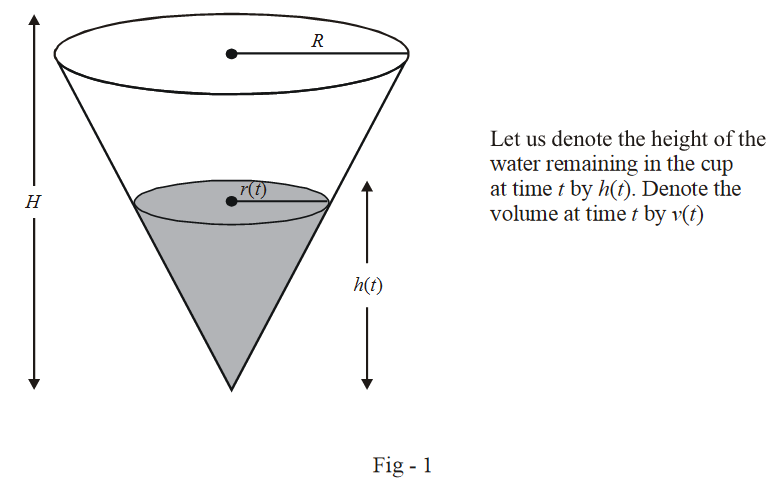# Differential Equations Set-2

Go back to  'SOLVED EXAMPLES'

Example – 3

A right circular cone with radius R and height H contains a liquid which evaporates at a rate proportional to its surface area in contact with air (proportionality constant = k > 0). Find the time after which the cone is empty.

Solution: We need to form a differential equation which describes the variation of the amount of water left in the cone with time.From the geometry described in the figure above,

\begin{align}& \qquad \frac{{r\left( t \right)}}{{h\left( t \right)}} = \frac{R}{H}\\& \Rightarrow \quad h\left( t \right) = \frac{H}{R}r\left( t \right)\end{align}

The volume v(t) of the cone is

\begin{align}&v\left( t \right) = \frac{1}{3}\pi \,{r^2}\left( t \right)h\left( t \right)\\& = \frac{{\pi \,H}}{{3R}}{r^3}\left( t \right) \qquad \qquad \qquad \ldots (1)\end{align}

Now, it is specified that the rate of evaporation (the rate of decrease of the water’s volume) is proportional to the surface area in contact with air:

$\frac{{dv}}{{dt}} = - k{r^2} \qquad \qquad \ldots (2)$

From (1) and (2), we have

\begin{align}& \qquad \frac{H}{R}{r^2}\frac{{dr}}{{dt}} = - k{r^2}\\& \Rightarrow \qquad \frac{{dr}}{{dt}} = - \frac{{Rk}}{H}\end{align}

This is the DE representing the variation in the radius of the water surface with time. The inital radius is R and the final radius is 0. If the time taken for the entire water to evaporate is T, we have

\begin{align}& \qquad \int\limits_R^0 {dr\,\, = - \,\,\frac{{Rk}}{H}} \,\int\limits_0^T {dt} \\ &\Rightarrow \qquad T = \frac{H}{k}\end{align}

Note that the time taken is independent of the radius of the cone and depends only on its height. Thus, for example, two cones full of water, with the same height, but one of them having a radius say a 1000 times larger than the other, will become empty in the same amount of time!

Example – 4

A curve C has the property that if the tangent drawn at any point P on C meets the coordinate axes at A and B, then P is the mid-point of AB. C passes through (1, 1). Determine its equation.

Solution: Let the curve be $$y = f\left( x \right).$$

The tangent at any point $$P\left( {x,y} \right)$$ has the equation

$Y - y = \frac{{dy}}{{dx}}\left( {X - x} \right)$

This meets the axes in $$A\left( {x - y\frac{{dx}}{{dy}},0} \right)$$ and $$B\left( {0,y - x\frac{{dy}}{{dx}}} \right).$$ Since P itself is the mid-point of AB,

we have

\begin{align} &\qquad x - y\frac{{dx}}{{dy}} = 2x,y - x\frac{{dy}}{{dx}} = 2y\\& \Rightarrow \quad \frac{{dy}}{{dx}} = - \frac{y}{x}\end{align}

This is in V.S form and can be solved by straight forward integration:

\begin{align}& \qquad \quad \int {\frac{{dy}}{y} = - } \int {\frac{{dx}}{x}} \\& \Rightarrow \qquad \ln y = - \ln x + \ln k\,\, & & \left( {k\,{\rm{is\;an\;arbitrary \;constant}}} \right)\\& \Rightarrow \qquad xy = k \end{align}

Since the curve C passes through (1, 1), we have k = 1. Thus, the equation of C is

$xy = 1$

Example – 5

A and B are two separate reservoirs of water. The capacity of reservoir A is double the capacity of reservoir B. Both the reservoirs are filled completely with water, their inlets are closed and then water is released simultaneuously from both the reservoirs. The rate of flow of water out of each reservoir at any instant of time is proportinal to the quantity of water in the reservoir at that time. One hour after the water is released, the quantity of water in reservoir A is $$1\frac{1}{2}$$ times the quantity of water in reservoir B. After how many hours do both the reservoirs have the same quantity of water?

Solution: Assume the intial volumes of water in A and B to be 2 V and V.

Denote the volume of water in A and B by $${v_1 }$$ and $${v_2}$$ respectively. We have,

$\frac{{d{v_1}}}{{dt}} = - {k_A}{v_1},\,\,\,\frac{{d{v_2}}}{{dt}} = - {k_B}{v_2}$

where $${k_A }$$ and $${k_B }$$ are constants of proportionality (not given). These two DEs are in VS form and the solution can be obtained by simple integration.

\begin{align} &\qquad \qquad \int\limits_{2V}^{{v_1}\left( t \right)} {\frac{{d{v_1}}}{{{v_1}}} = \int\limits_0^t { - {k_A}} dt} \,,\,\,\,\,\,\,\int\limits_V^{{v_2}\left( t \right)} {\frac{{d{v_2}}}{{{v_2}}}} = \,\,\,\int\limits_0^t { - {k_B}} \,dt\\ &\Rightarrow \qquad \ln \frac{{{v_1}\left( t \right)}}{{2V}} = - {k_A}t, \quad \ln \frac{{{v_2}\left( t \right)}}{V} = - {k_B}t\\&\Rightarrow \qquad {v_1}\left( t \right) = 2V{e^{ - {k_A}t}}, \quad{v_2}\left( t \right) = V{e^{ - {k_B}t}}\end{align}

It is given that

\begin{align} &\qquad\quad {v_1}\left( {t = 1} \right) = \frac{3}{2}{v_2}\left( {t = 1} \right)\\ &\Rightarrow \quad 2V{e^{ - {k_A}}} = \frac{3}{2}V{e^{{k_B}}}\\& \Rightarrow \quad {e^{\left( {{k_B} - {k_A}} \right)}} = \frac{3}{4}\\ & \Rightarrow \quad {k_B} - {k_A} = \ln \left( {\frac{3}{4}} \right)\end{align}

Let T be the time at which the volumes in the two reservoirs become equal. We thus have,

\begin{align}& {v_1}\left( {t = T} \right) = {v_2}\left( {t = T} \right)\\ \Rightarrow \qquad &2V{e^{ - {k_A}T}} = V{e^{ - {k_B}T}}\\ \Rightarrow \qquad &{e^{\left( {{k_A} - {k_B}} \right)T}} = 2\\ \Rightarrow \qquad &\left( {{k_A} - {k_B}} \right)T = \ln 2\\ \Rightarrow \qquad &T\ln \left( {\frac{4}{3}} \right) = \ln 2\\ \Rightarrow \qquad &T = \frac{{\ln 2}}{{\ln \left( {4/3} \right)}}\,\,\,\,\,\,\,{\rm{hours}}\end{align}

Learn from the best math teachers and top your exams

• Live one on one classroom and doubt clearing
• Practice worksheets in and after class for conceptual clarity
• Personalized curriculum to keep up with school